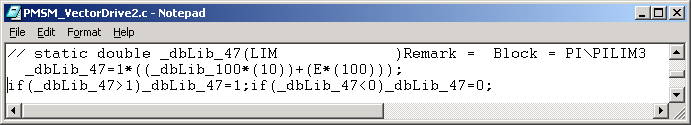• Introduction
• What is in this manual
• What is Caspoc
• User interface
• Introduction
• Starting
• Simulation
• Editing
• Viewing and printing
• Getting Started
• Basic editing
• Simulation in the time domain
• Basic User Interface Topics
• Editing
• Simulation
• Viewing
• Library
• Reports
• Project management
• Circuit and Block Diagram Components
• Introduction
• Cscript and user defined functions
• Component parameters
• Modeling Topics
• Introduction
• Power Electronics
• Semiconductors
• Electrical Machines
• Electrical drives
• Power Systems
• Mechanical Systems
• Thermal Systems
• Magnetic Circuits
• Green Energy
• Coupling to FEM
• Experimenter
• Analog hardware description language
• Embedded C code Export
• Coupling to Spice
• Small Signal Analysis
• Matlab coupling
• Tips and tricks
• Appendices

## Permanent Magnet Synchronous Machine Vector Drive

A Permanent Magnet Synchronous Machine (PMSM) is used in a Vector Drive. In this example, the power electronics inverter, the PMSM, the mechanical load and the vector control are modeled.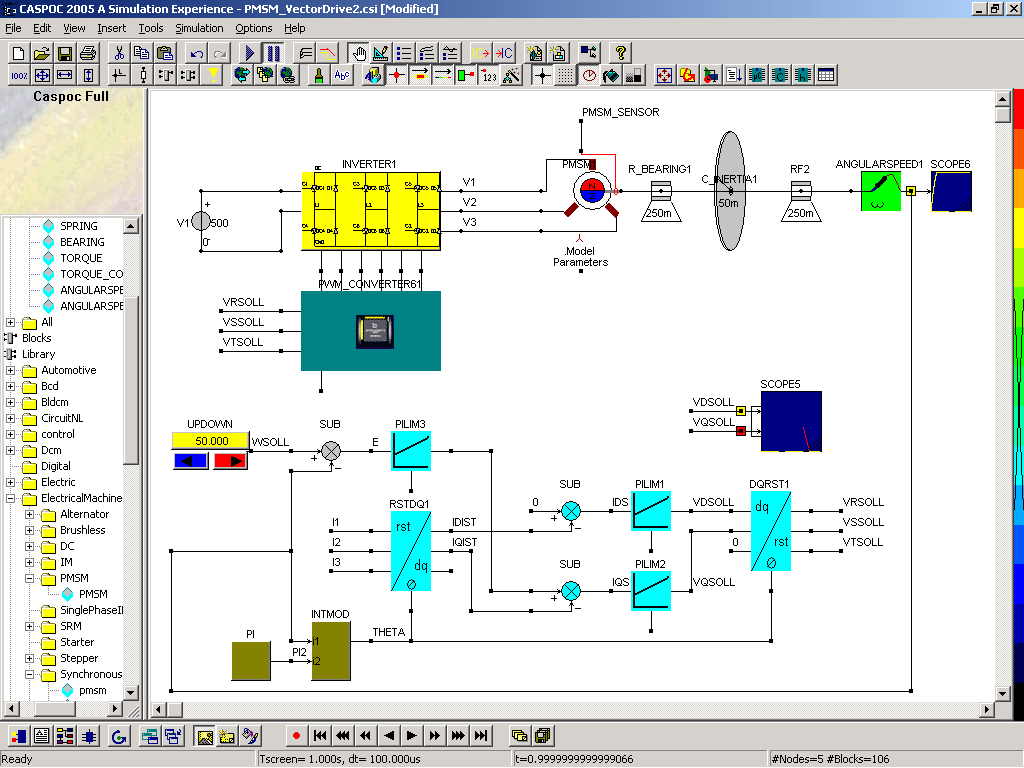The Permanent Magnet Synchronous Machine (PMSM) is used in this vector controlled application. An inverter is connected to the PMSM. The model for the PMSM is a dq model of the synchronous machine with permanent magnet excitation. The torque produced by the PMSM is connected to a mechanical drive train, being a rotating mass with to bearings. The inertia of the rotating mass is 0.05 [kgm2] and the total friction of the bearings is 0.5 [Nms/Rad]. A sensor measures the angular speed of the shaft and using the INTMOD block, the position of the shaft is calculated with a modulus of 2*π.

The stator currents are measured using current sensors in the PMSM. From these currents i1, i2, i3, the stator currents in the DQ frame IDIST and IQIST are derived, using the position of the shaft THETA.

The measured values of the stator current in the DQ frame are compared with the set values in the DQ frame and the resulting and the resulting vector (IDS, IQS) is controls the required stator voltage In the DQ frame VDSOLL and VQSOLL using two PI controllers.

The preset value of the current on the direct axis is set to 0. The speed controller PILIM3 controls the preset value on the quadrature axis. The speed controller is build from one PI controller that has as input the error signal between the measured and preset angular speed and the output is the required stator current on the quadrature axis.

The controlled stator voltage in the DQ frame is transformed into the real stator voltage (VRSOLL, VSSOLL, VTSOLL) that is input to the pulse width modulator.

After starting the simulation, the angular speed of the shaft can be controlled by clicking the animated UPDOWN control block in the simulation. The simulation will continue and show the response to the new preset value for the angular speed.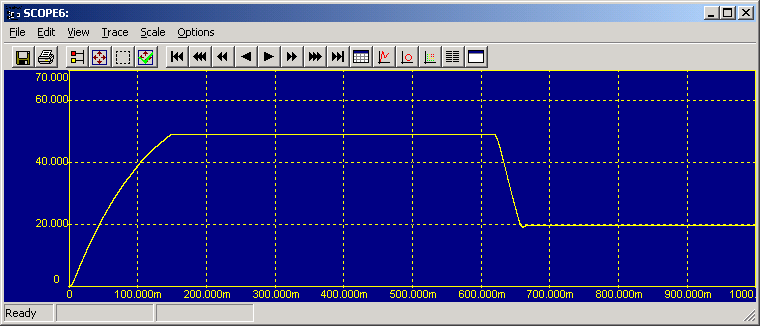During the simulation the internal structure of the converter can be viewed and even the current flow in the inverter can be followed using the animation feature. In the project manager on the left side click on Project/Schematic and click the library block that you want to view. In this case the library block "INVERTER1".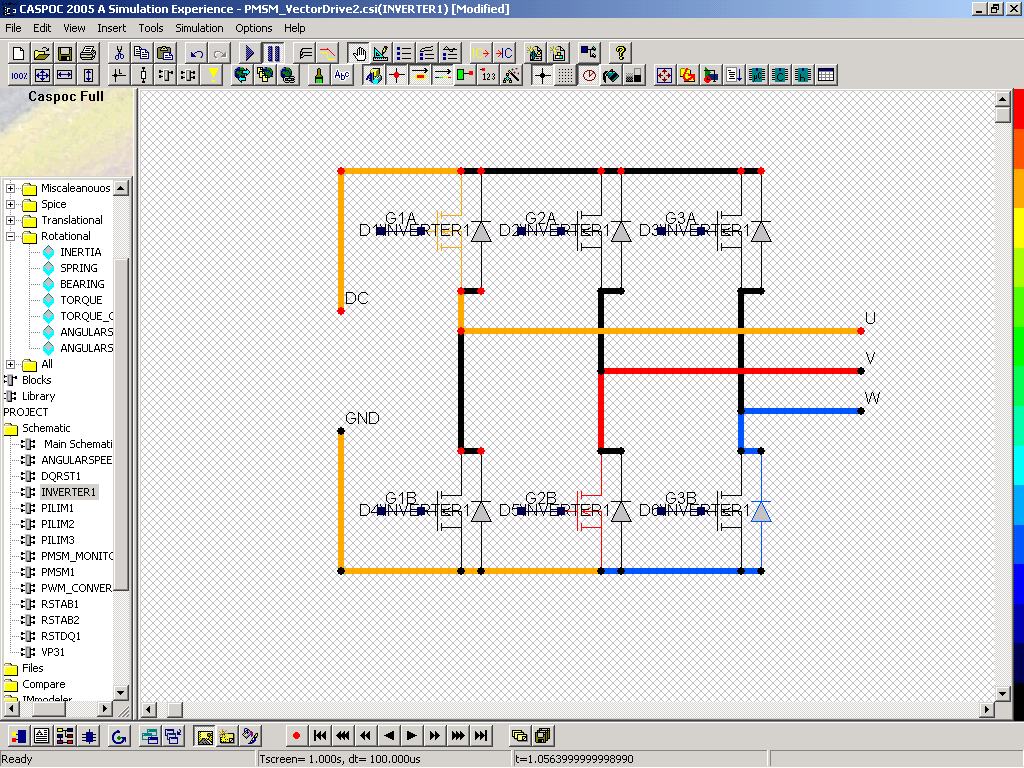The animation of the currents in the schematic show that at this certain point in time (when the screenshot was taken), phase U is connected to the positive DC link terminal via the orange colored mosfet. On the lower side there is also a freewheeling process taking place, via the bottom right diode (blue) and its neighboring mosfet (red).

To view the working of other library blocks, simply click the name of the library block in the project manager, for example the RST to DQ transformation.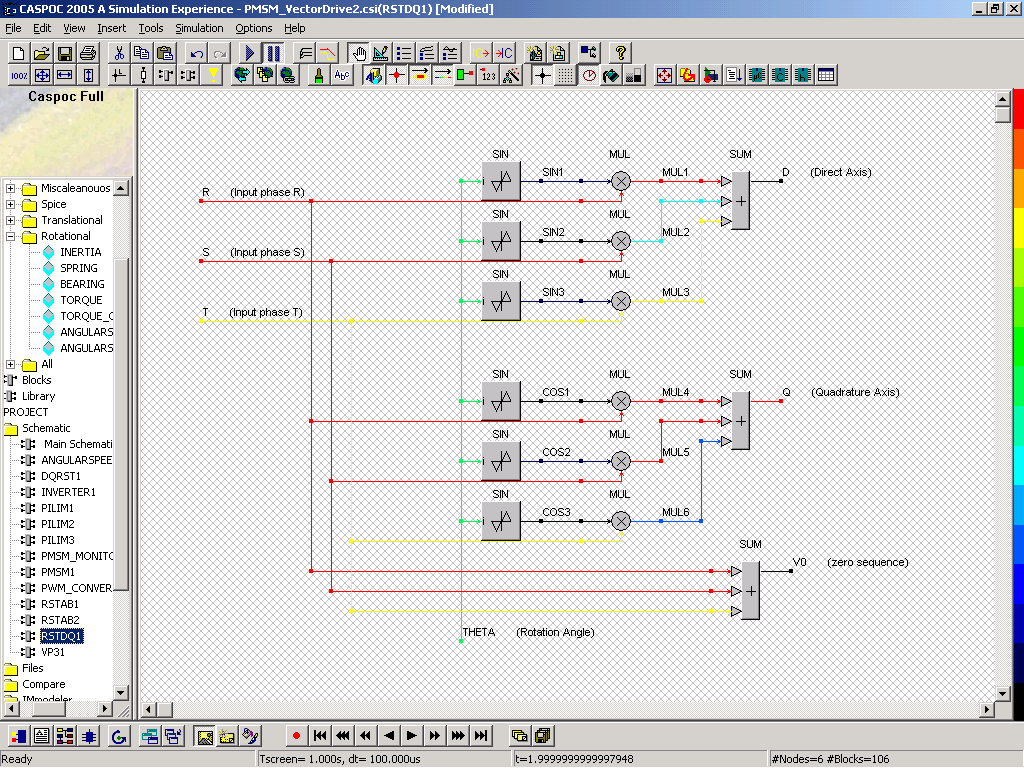From the control in he block diagram the C code can be exported and used in a DSP or microcontroller.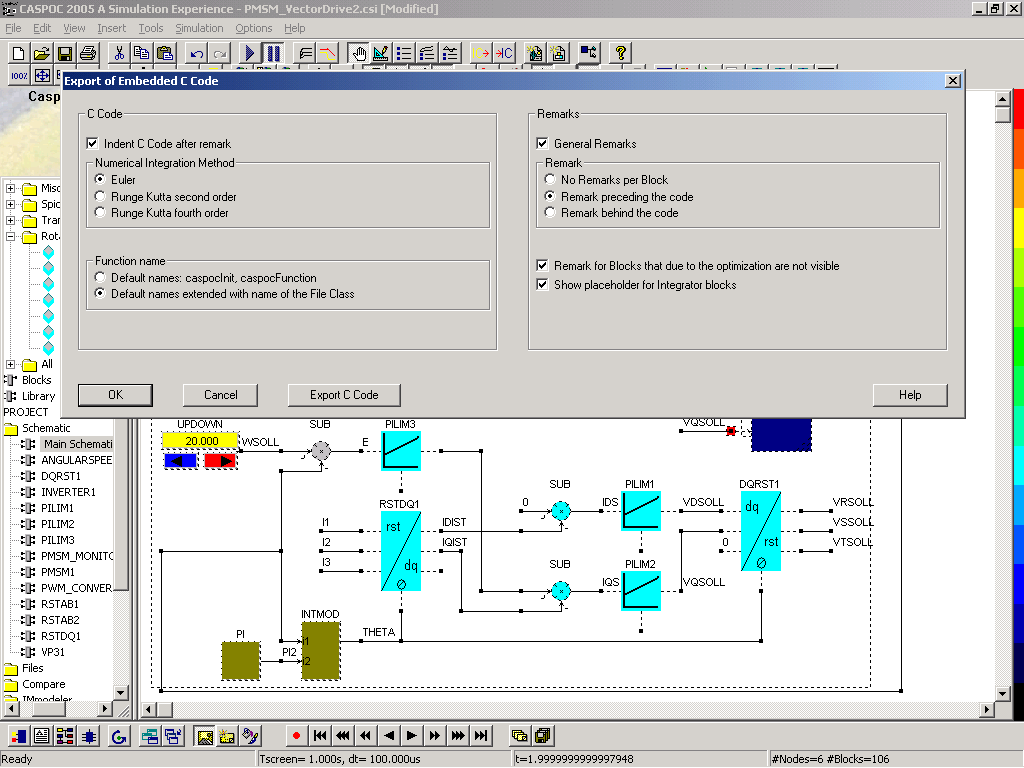After exporting the C code, the C code can be viewed directly from Caspoc or included into your main embedded C project.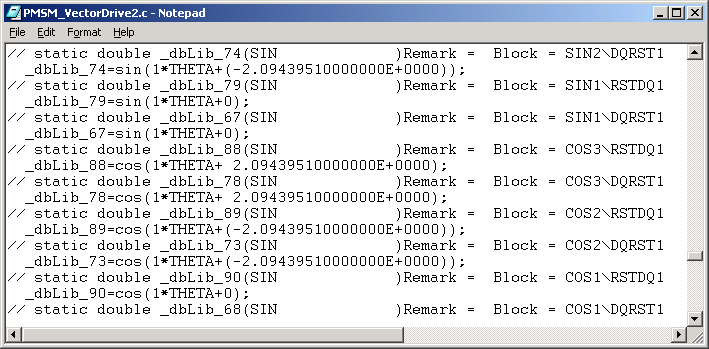The exported code is optimized for execution speed and not used block names are optimized, to condense the exported C code.